Online Tution   »   Education News   »   CBSE Class 10 Maths Term 2...

# CBSE Class 10th Maths Term 2 Answer Key & Paper Analysis 2022

## Class 10 Maths Term 2 Paper Analysis 2022

CBSE Class 10 Mathematics Term 2 Exam Analysis 2022: The board will conduct CBSE Class 10 Term 2 Mathematics Exam 2022 today, 5th May 2022. The CBSE Class 10 Term 2 Mathematics Exam 2022 will begin at 10:30 am and conclude at 12:30 pm. After examination, the students will rush to find the CBSE Class 10 Mathematics Term 2 Exam Analysis 2022. To aid students, we have provided the detailed CBSE Class 10 Mathematics Term 2 Exam Analysis 2022 on this page. Read the CBSE Class 10 Mathematics Term 2 Exam Analysis 2022 and bookmark this page to get CBSE Class 10 Term 2 Exam Analysis 2022 for other subjects.

## CBSE Class 10 Maths Term 2 Exam Analysis 2022: Overview

Here we have provided the miscellaneous information regarding the CBSE Class 10 Mathematics Term 2 Exam Analysis 2022. The students appearing in the CBSE Class 10 Term 2 Exam 2022 must check the information provided in the table below:

 CBSE Class 10 Mathematics Term 2 Exam Analysis 2022 Exam Conducting Body Central Board of Secondary Education Exam & Subject Name CBSE Class 10 Mathematics Category Exam Analysis Exam Date 5th May 2022 Exam Time 10:30 am to 12:30 pm Official Answer Key To be notified Official Website https://www.cbse.nic.in/

## CBSE Class 10 Mathematics Term 2 Exam Analysis 2022: Pattern

The CBSE Class 10 Term 2 Mathematics question paper consists of three sections namely Section A, Section B, and Section C. Check the other details listed below:

CBSE Class 10 Term 2 Basic Maths Exam pattern: The basic maths question paper consists of 14 marks. Check the section-wise details listed below

1. Section A: In this section 6 questions of 2 marks each will be asked.
2. Section B: In this section 4 questions of 3 marks each will be asked.
3. Section C: In this section 4 questions of 4 marks each will be asked.

CBSE Class 10 Term 2 Standard Maths Exam pattern: The standard maths question paper consists of 14 marks. Check the section-wise details listed below

1. Section A: In this section 6 questions of 2 marks each will be asked.
2. Section B: In this section 4 questions of 3 marks each will be asked.
3. Section C: In this section 4 questions of 4 marks each will be asked.

## CBSE Class 10 Mathematics Term 2 Exam Analysis 2022

1. The students who appeared in CBSE Class 10 Term 2 Mathematics Exam 2022 found the paper lengthy and of moderate difficulty level.
2. As per the experts, the Section A questions were quite easy, the students will easily score the full marks if they have attempted the paper well.
3. In Section B, 1 or 2 questions has troubled students.
4. The case studies asked in Section C was quite easy. with proper conceptual understanding the students can easily score the good marks in case studies.
5. The Basic maths paper was quite easy than standard maths.
6. The experts has concluded that the paper was moderate but was time consuming. Only students who have completed the whole syllabus can attempt the whole syllabus in given time.

Related Post:

## CBSE Class 10 Mathematics Term 2 Exam Analysis 2022: FAQs

Q. What is the CBSE Class 10 Mathematics Term 2 Exam Analysis 2022?

On this page, we have provided the CBSE Class 10 Mathematics Term 2 Exam Analysis 2022. In CBSE Class 10 Mathematics Term 2 Exam Analysis 2022, we have provided complete information regarding the level of the exam.

Q. What is the subject code of CBSE Class 10 Mathematics?

The subject code of CBSE Class 10 Basic Maths is 241 and Mathematics Standard is 041.

Q. What is the exam time for CBSE Class 10 Mathematics?

The CBSE Class 10 Term 2 Mathematics Examination will be held on 5th May  2022 between 10:30 am to 12:30 pm.

Sharing is caring!

### TOPICS:

•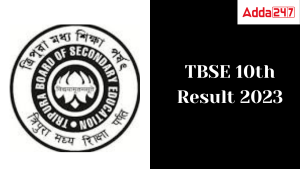TBSE Madhyamik Result 2023 Date, Tripura...
•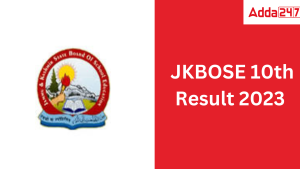JKBOSE 10th Result 2023 Date, JK Board C...
•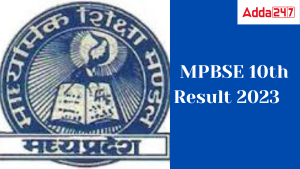MP Board 10th Result 2023 Link Out, MP 1...
•UK Board Result 2023 Class 10 Out, Direc...
•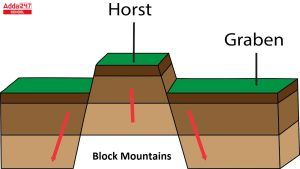Block Mountains: Examples Types, Format...
•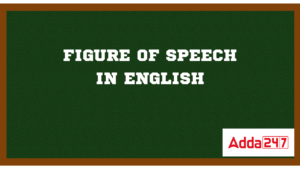Figures of Speech in English with Exampl...